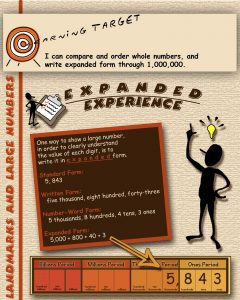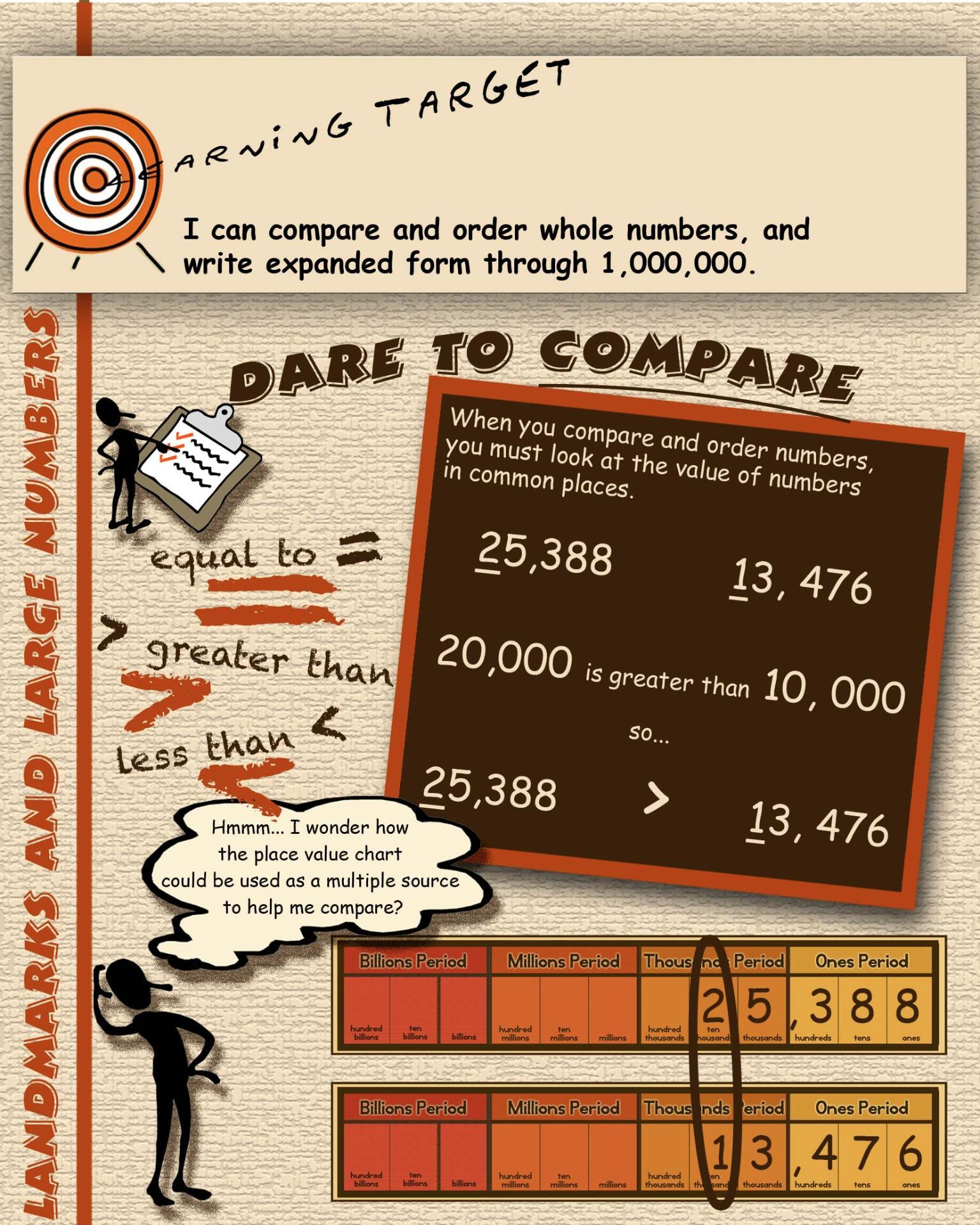# 1 Place Value, Number Sense, Addition, and Subtraction

Below you will find detailed information about each unit we will cover in this course. New information will be added as necessary so please check back.

Math Unit 1: Place Value, Number Sense, Addition, & Subtraction

Big Idea/Essential Questions:

1. How do units within a system relate to each other?
2. How are place value patterns repeated in larger numbers?
3. How can I use words and expanded formats to order and compare numbers?
4. How can I justify when estimation is more appropriate than finding an exact answer?
5. What are the strategies I use to find sums and differences of numbers?

Intended Learning Outcomes:

• I can relate the dimensions of a rectangle to addition (repeated addition)
• I can demonstrate an understanding of the process of addition with regrouping.
• I can demonstrate an understanding of the process of subtraction with regrouping.
• I can know and fluently use addition and subtraction facts.
• I can add lists of numbers.
• I can add numbers to the millions.
• I can apply the commutative and associative properties.
• I can subtract numbers to the millions.
• I can round numbers to the nearest place value to the millions.
• I can find the perimeter of a rectangle.
• I can solve and pose one-step and multi-step story problems.
• I can draw a labeled sketch or diagram to represent a story problem or mathematical situation.

Key Vocabulary:

minuend          subtrahend         difference

place value        round         estimate        compare

commutative       associative

perimeter